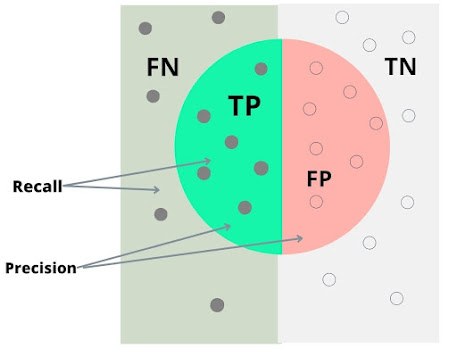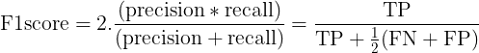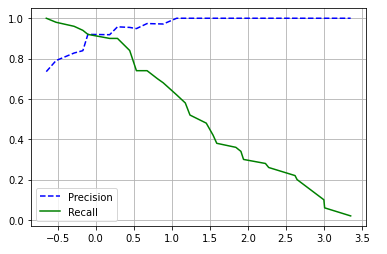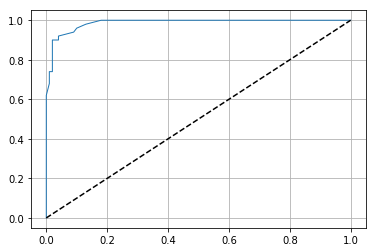# Precision and Recall in Classification: Definition, Formula, with Examples

This article will give an in depth understanding of precision,recall, ROC curve,f1-score. why these are important? and basic definition with examples.

When you’re evaluating the results of your classification project, there are two very important accuracy measures to consider that go beyond the standard hit rate: precision and recall. Why are these measures really important in classification? Well, Precision gives you an idea of how accurate your classifications are, and it answers the question what percentage of my positive results are actually positive? Recall tells you how good your classifications really are, and it answers the question what percentage of relevant results do I get? When you evaluate a machine learning algorithm, you need to keep both of these accuracy measures in mind so that you can find the best balance between them.

Precision, Recall, ROC curve, and Confusion matrix are some of the performance measures in classification problems. Among them, Precision and Recall play an important role to measure how well a classifier is performing on a set of test data. In general, there is no single metric that can be used to evaluate all classifiers under all circumstances. However, precision and recall are one such metrics that can be used to evaluate different classifiers under different conditions. In addition to these two metrics, another useful metric is the ROC curve which gives us a graphical view of how well our classifier performs at identifying positives as compared to negatives. This post will discuss these three metrics in detail along with an example using the Iris dataset from sklearn library using python.

## Definition of Precision and Recall

The precision is basically defined as the ratio of correctly predicted positive classes to all predicted positive classes. It can be expressed mathematically as:

Precision = TP / (TP + FP) (where TP is True Positives and FP is False Positives)

The recall is simply defined as a ratio of correctly predicted positive classes to all actually existing positive classes. It can be expressed mathematically as:

Recall = TP / (TP + FN) (where FN is False Negative)Precision-Recall Demonstration

The ROC curve (Receiver Operating Characteristic) as we said earlier is based on these two measures, but it also includes a third measure, which adds up both values (recall + precision).In other words, you get an AUC(Area under the curve) value between 0 and 1. A value closer to 1 means that your model does better in predicting positives than negatives. An AUC value closer to 0 means that your model does worse than random chance at predicting positives or negatives.

## Examples of Precision and Recall

Let's look at some examples for precision and recall, using a binary classification problem. For example, let's say we have a machine learning algorithm that is used to identify spam emails. We might have a dataset of emails that are known to be spam (the positive set) and another dataset of emails that are known not to be spam (the negative set). If our machine learning algorithm has an accuracy score of 90%, it means that out of all emails in our positive set, 90% were correctly identified as spam. Similarly, if it has an accuracy score of 10%, it means that out of all emails in our negative set, 10% were incorrectly identified as spam.

So the precision here means that 90% of all emails in our positive set were correctly classified as spam, while recall means that only 10% of all emails in our negative set were incorrectly classified as spam. In other words, Precision = True Positives / (True Positives + False Negatives), while Recall = True Positives / (True Positives + False Negatives + False Positives). What do you think? Is one better than the other? Is there a way to combine them into one metric? yes, the f1-score.

## The f1-score

Combining precision and recall into a single metric is known as the f1-score. It’s simply (precision * recall) / (precision + recall). It’s also sometimes called f-score. If you have an accuracy of 75%, your f1 score will be 0.75 * 0.75 = 0.5625, which means that 56% of your predictions are correct. This number can be interpreted like any other accuracy measure—the higher it is, the better.Equation for F1-score

## The ROC curve

ROC stands for receiver operating characteristic. It's a standardized way of measuring how good a classification model is at discriminating between two things, like whether an email is a spam or not.  ROC curves plot true positive rate (TPR) against false positive rate (FPR).  TPR measures the rate of how often you correctly identify something as belonging to a certain class; FPR measures the rate of how often you incorrectly identify something as belonging to that class. If your curve has a high area under it, then your model has a high precision (low FPR) and recall (high TPR). If your curve has a low area under it, then your model has low precision and recall.

## Precision-Recall Graph

You can think of these as hit rate versus miss rate or true positive rate versus false-positive rate. Typically, you want to tune your classifier to maximize either precision or recall but not both at once (maximizing one will necessarily make your other number smaller). For example, if you are classifying emails into spam/not spam (yes) then a high precision value might be good (however, it may also mean that lots of false positives are being marked as spam). By contrast, a low recall value is bad since it means that you could be missing some truly spam emails.

Increasing precision reduces recall and vice versa. This is called the precision/recall tradeoff.

In fact, precision/recall curves can help you find a better threshold value. Precision is plotted on the x-axis, while recall is plotted on the y-axis. As such, when recall increases at a given precision, it moves up along an upward sloping line with a positive slope. Similarly, as precision increases with a given recall, it moves right along an upward sloping line with a negative slope. A point on either of these lines gives us a tradeoff between precision and recall, which we call a balanced F1 score. A balanced F1 score is one that has an equal distance from both the precision and recall axis. For example, if we have a balanced F1 score of 0.5 then there are an equal number of true positives (TP) and false positives (FP). A balanced F1 score can be achieved by increasing either precision or recall. But if we increase both then the balanced F1 score will decrease again because of the precision/recall tradeoff.

## Example using Iris dataset

Now let's do an example to make these measures clear, We are using the Iris-dataset for this example. If you are not familiar with Iris-dataset and its classification, check this article: Iris-dataset classification: A tutorial .

The first thing we need to do is to import the Iris dataset from sklearn

from sklearn.datasets import load_irisiris = load_iris()iris.keys()dict_keys(['data', 'target', 'frame', 'target_names', 'DESCR', 'feature_names', 'filename', 'data_module'])

Let's create our train and test set using sklearn train_test_split method.

from sklearn.model_selection import train_test_splitimport numpy as npX = iris["data"][:, 3:] # petal widthy = (iris["target"] == 2).astype(np.int) # 1 if Iris-virganica, else 0X_train, X_test, y_train, y_test = train_test_split(X,y, test_size=0.7, random_state=42)

Note that we are performing a binary classification here, considering the two cases, whether the flower is Iris-virginica or not based on their petal lengths and widths. So here, our y set contains two classes(whether the flower is Iris-virginica(1) or not(0))

Importing the Logistic Regression model,

from sklearn.linear_model import LogisticRegressionlog_reg = LogisticRegression()log_reg.fit(X_train,y_train)

### Making predictions and evaluating the classifier

Let's do the predictions and see what will be our precision, recall, and, f1score

test_predictions = log_reg.predict(X_test)from sklearn.metrics import precision_score, recall_score, f1_score, confusion_matrixprecision = precision_score(y_test, test_predictions)recall = recall_score(y_test, test_predictions)f1_score_ = f1_score(y_test, test_predictions)confusion_matrix_ = confusion_matrix(y_test, test_predictions)print("Precision:", precision)print("Recall:",recall)print("F1-Score:", f1_score_)print("\nConfusion matrix")print(confusion_matrix_)output:Precision: 0.9354838709677419Recall: 0.90625F1-Score: 0.9206349206349206Confusion matrix[[71  2] [ 3 29]]

What we can understand from the results? you can see that the precision is greater than 93% which means the model is able to predict 93% of Iris-virginica from the total number of Iris-virginica species. However, recall is about 90% means that the model is able to recognize 90% of the Iris-Virginica from the overall dataset.

Let's now plot the precision-recall curve and ROC curve using matplotlib, But before that, we need to perform cross-validation in order to find the precision and recall in different cases, let's see how this can be done.

from sklearn.metrics import precision_recall_curvefrom sklearn.model_selection import cross_val_predicty_scores = cross_val_predict(log_reg, X, y, cv = 3, method="decision_function")precisions, recalls, thresholds = precision_recall_curve(y, y_scores)

What happens here is that the cross_val_predict method will perform repeated predictions using the Logistic Model and then these scores are passed to precision_recall_curve for finding the precision-recall distribution for a given threshold.

When we plot the curve using matplotlib

import matplotlib.pyplot as pltplt.plot(thresholds, precisions[:-1], "b--", label="Precision")plt.plot(thresholds, recalls[:-1], "g-", label="Recall")plt.legend(loc='best')plt.grid()

We'll get this:Precision-Recall curve for given data

Now let's plot the ROC curve,

from sklearn.metrics import roc_curvefpr, tpr, thresholds = roc_curve(y, y_scores)plt.plot(fpr, tpr, linewidth=1)plt.plot([0, 1], [0, 1], 'k--')plt.grid()plt.show()ROC curve for given data

The dotted line represents the ROC curve of a random classifier, A good classifier will deviate more from the dotted line towards the top-left. Another way to measure the classifier using the ROC curve is to look at the Area Under The Curve(AUC). The AUC increase relative to the performance of the classifier, ie, A super perfect 100% accuracy model has a ROC curve of 1.

You may also like

Iris-dataset classification using python

Concept of Logistic Regression

Multinomial Logistic Regression for classification